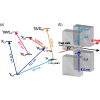Physical Review Letters ( IF 8.385 ) Pub Date : 2020-10-16 , DOI: 10.1103/physrevlett.125.163001
H. A. Fürst; C.-H. Yeh; D. Kalincev; A. P. Kulosa; L. S. Dreissen; R. Lange; E. Benkler; N. Huntemann; E. Peik; T. E. MehlstäublerWe report on the first coherent excitation of the highly forbidden ${{}^{2}S}_{1/2}\to {{}^{2}F}_{7/2}$ electric octupole (E3) transition in a single trapped ${}^{172}{\mathrm{Yb}}^{+}$ ion, an isotope without nuclear spin. Using the transition in ${}^{171}{\mathrm{Yb}}^{+}$ as a reference, we determine the transition frequency to be 642 116 784 950 887.6(2.4) Hz. We map out the magnetic field environment using the forbidden ${{}^{2}S}_{1/2}\to {{}^{2}D}_{5/2}$ electric quadrupole (E2) transition and determine its frequency to be 729 476 867 027 206.8(4.4) Hz. Our results are a factor of $1×{10}^{5}$ ($3×{10}^{5}$) more accurate for the E2 (E3) transition compared to previous measurements. The results open up the way to search for new physics via precise isotope shift measurements and improved tests of local Lorentz invariance using the metastable ${{}^{2}F}_{7/2}$ state of ${\mathrm{Yb}}^{+}$.

down
wechat
bug